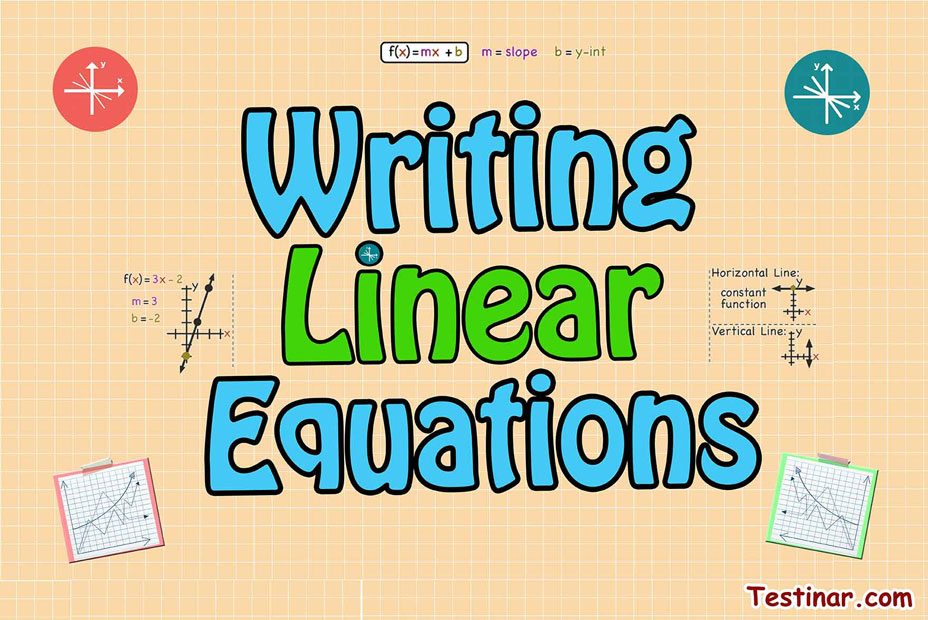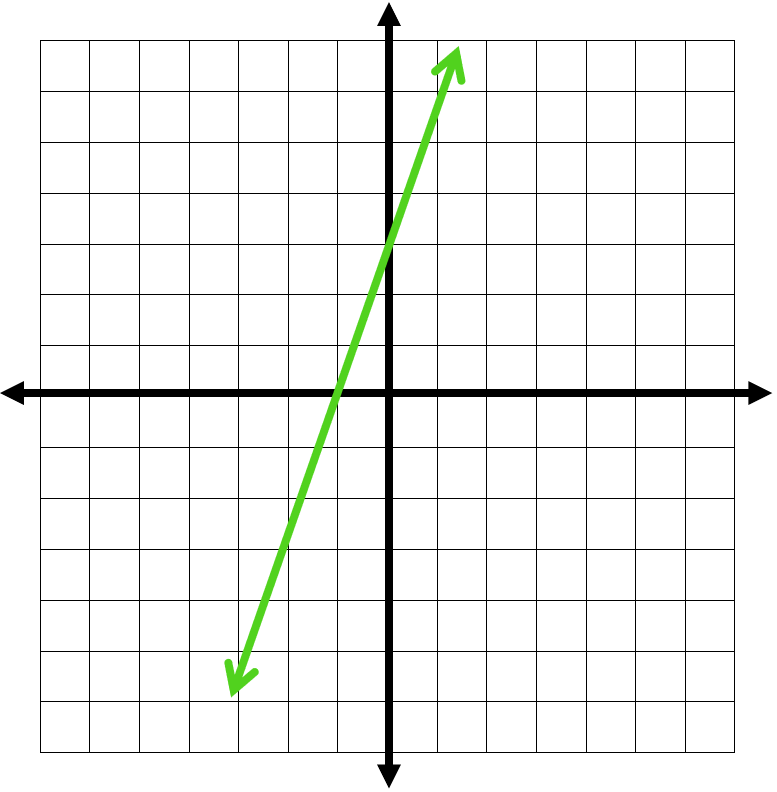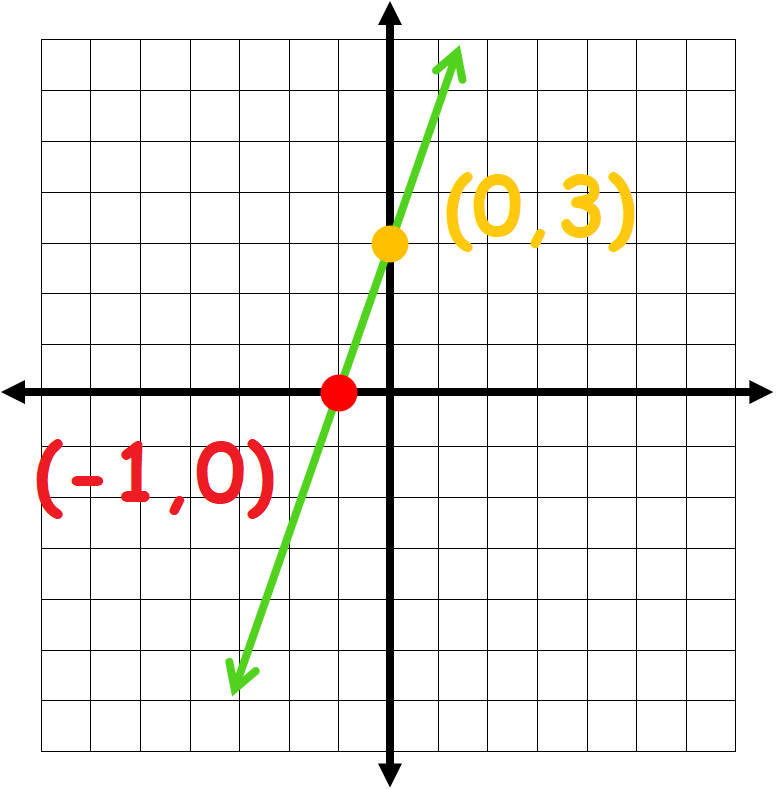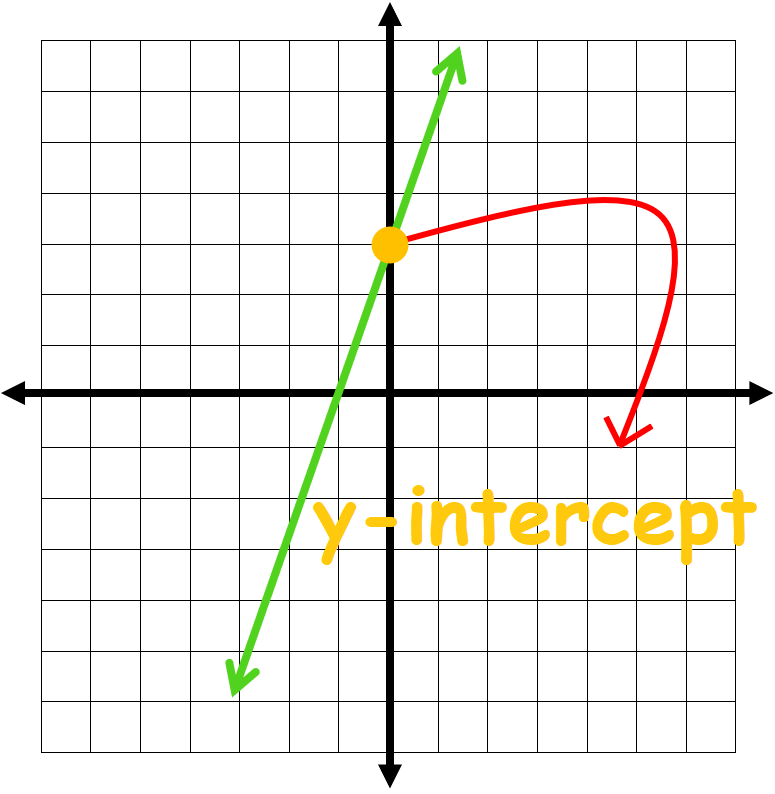## How to Write Linear Equations?

An equation presented in slope-intercept form is written as $$y \ =\ mx \ + \ b$$
Where $$m$$ is the slope of the line and $$b$$ is the $$y$$-intercept. If you know the slope and the $$y$$-intercept, you can use this equation to write a line equation.

### Example

Find the equation of the line that passes through the points $$(-1,0)$$  and $$(0,3)$$ .Solution:

We should find the $$b$$-value and the $$y$$-intercept, to do that, so we select two points on the line:Now, determine the slope between the $$2$$ points:

$$m \ = \ \frac{y_2 \ - \ y_1}{x_2 \ - \ x_1} \ = \ \frac{0 \ - \ 3}{-1 \ - \ 0} \ = \ \frac{-3}{-1} \ = \ 3$$

The $$b$$-value can be found by just looking at the graph:$$⇒ \ b \ = \ 3$$

Now, we can use the equation $$y \ =\ mx \ + \ b$$ and write the line's equation:

$$y \ =\ mx \ + \ b \ ⇒ \ y \ =\ 3x \ + \ 3$$

In a lot of cases, the value of $$b$$ isn’t read so easily. In that case, or in cases where you are not sure if the line really crosses the $$y$$-intercept at this particular point, $$b$$ can be calculated by solving the equation for $$b$$, then substituting $$x$$ and $$y$$ with one of the two points.

The previous example can be used to explain this:

• The two points are $$(-1 \ , \ 0)$$, and $$(0 \ , \ 3)$$. We determined the slope from these two points: $$m \ = \ 3$$
• Now we have the equation: $$y \ = \ 3x \ + \ b$$
From that the equation can be solved for $$b$$: $$b \ = \ y \ - \ 3x$$
• Also, if we input the values from the second point $$(0 \ , \ 3)$$, we have: $$b \ = \ 3 \ - \ 3(0) \ = \ 3$$
• Finally, we can write the line equation: $$y \ = \ 3x \ + 3$$

### Summary:

Writing linear equations:

• Step1: Determine the slope, $$m$$. This can be done by utilizing the slope formula to get the slope between two known points on the line.
• Step2: Determine the $$y$$-intercept. In order to do this, simply substitute the slope and the $$(x \ , \ y)$$ coordinates of a point on the line in the slope-intercept formula and then calculate $$b$$.
• Step3: Once you have $$m$$ and $$b$$, you can enter them into the slope-intercept equation and find the line equation.

### Exercises for Writing Linear Equations

1) Find the line equation of the line passing through $$(10,9)(11,0)$$$$\ \Rightarrow \$$

2) Find the line equation of the line passing through $$(8,7)(9,-5)$$$$\ \Rightarrow \$$

3) Find the line equation of the line passing through $$(7,10)(8,-6)$$$$\ \Rightarrow \$$

4) Find the line equation of the line passing through $$(9,5)(10,3)$$$$\ \Rightarrow \$$

5) Find the line equation of the line passing through $$(10,2)(11,3)$$$$\ \Rightarrow \$$

6) Find the line equation of the line passing through $$(1,4)(2,2)$$$$\ \Rightarrow \$$

7) Find the line equation of the line passing through $$(7,3)(8,5)$$$$\ \Rightarrow \$$

8) Find the line equation of the line passing through $$(2,9)(3,5)$$$$\ \Rightarrow \$$

9) Find the line equation of the line passing through $$(3,6)(4,-4)$$$$\ \Rightarrow \$$

10) Find the line equation of the line passing through $$(5,1)(6,-3)$$$$\ \Rightarrow \$$

1) Find the line equation of the line passing through $$(10,9)(11,0)$$$$\ \Rightarrow \ \color{red}{m = \frac{0 \ - \ 9}{11 \ - \ 10} = -9}$$$$\ \Rightarrow \ \color{red}{y - 9= m(x - 10)}$$$$\ \Rightarrow \ \color{red}{y = -9x \ + \ 99}$$
2) Find the line equation of the line passing through $$(8,7)(9,-5)$$$$\ \Rightarrow \ \color{red}{m = \frac{-5 \ - \ 7}{9 \ - \ 8} = -12}$$$$\ \Rightarrow \ \color{red}{y - 7= m(x - 8)}$$$$\ \Rightarrow \ \color{red}{y = -12x \ + \ 103}$$
3) Find the line equation of the line passing through $$(7,10)(8,-6)$$$$\ \Rightarrow \ \color{red}{m = \frac{-6 \ - \ 10}{8 \ - \ 7} = -16}$$$$\ \Rightarrow \ \color{red}{y - 10= m(x - 7)}$$$$\ \Rightarrow \ \color{red}{y = -16x \ + \ 122}$$
4) Find the line equation of the line passing through $$(9,5)(10,3)$$$$\ \Rightarrow \ \color{red}{m = \frac{3 \ - \ 5}{10 \ - \ 9} = -2}$$$$\ \Rightarrow \ \color{red}{y - 5= m(x - 9)}$$$$\ \Rightarrow \ \color{red}{y = -2x \ + \ 23}$$
5) Find the line equation of the line passing through $$(10,2)(11,3)$$$$\ \Rightarrow \ \color{red}{m = \frac{3 \ - \ 2}{11 \ - \ 10} = 1}$$$$\ \Rightarrow \ \color{red}{y - 2= m(x - 10)}$$$$\ \Rightarrow \ \color{red}{y = x \ - \ 8}$$
6) Find the line equation of the line passing through $$(1,4)(2,2)$$$$\ \Rightarrow \ \color{red}{m = \frac{2 \ - \ 4}{2 \ - \ 1} = -2}$$$$\ \Rightarrow \ \color{red}{y - 4= m(x - 1)}$$$$\ \Rightarrow \ \color{red}{y = -2x \ + \ 6}$$
7) Find the line equation of the line passing through $$(7,3)(8,5)$$$$\ \Rightarrow \ \color{red}{m = \frac{5 \ - \ 3}{8 \ - \ 7} = 2}$$$$\ \Rightarrow \ \color{red}{y - 3= m(x - 7)}$$$$\ \Rightarrow \ \color{red}{y = 2x \ - \ 11}$$
8) Find the line equation of the line passing through $$(2,9)(3,5)$$$$\ \Rightarrow \ \color{red}{m = \frac{5 \ - \ 9}{3 \ - \ 2} = -4}$$$$\ \Rightarrow \ \color{red}{y - 9= m(x - 2)}$$$$\ \Rightarrow \ \color{red}{y = -4x \ + \ 17}$$
9) Find the line equation of the line passing through $$(3,6)(4,-4)$$$$\ \Rightarrow \ \color{red}{m = \frac{-4 \ - \ 6}{4 \ - \ 3} = -10}$$$$\ \Rightarrow \ \color{red}{y - 6= m(x - 3)}$$$$\ \Rightarrow \ \color{red}{y = -10x \ + \ 36}$$
10) Find the line equation of the line passing through $$(5,1)(6,-3)$$$$\ \Rightarrow \ \color{red}{m = \frac{-3 \ - \ 1}{6 \ - \ 5} = -4}$$$$\ \Rightarrow \ \color{red}{y - 1= m(x - 5)}$$$$\ \Rightarrow \ \color{red}{y = -4x \ + \ 21}$$

## Writing Linear Equations Quiz

### Ace the ISEE Middle LEVEL Math in 30 Days

$18.99$13.99

### 5 Full Length SSAT Middle Math Practice Tests

$11.99$15.99

### ISEE Upper Level Math Workbook 2020 – 2021

$21.99$16.99

### ISEE Middle Level Mathematics Prep 2019

$17.99$12.99